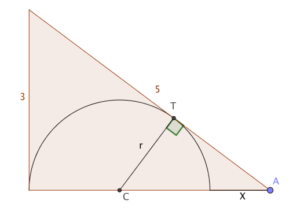A nice puzzle this week, via NRICH’s magnificent @ajk44: a semicircle is inscribed in a 3-4-5 triangle as shown. Find $X$.I think it’s a nice puzzle because Alison’s way of doing it was entirely different to mine, but thankfully got the same answer. You might like to try it yourself.

### My way

My way was to consider similar triangles. Draw a perpendicular at the point of tangency, T; that crosses the ‘4’ side at the centre, C. Now, the triangle ATC is similar to the original triangle (it also has a right angle and shares the angle at A, so all three angles are the same.)In particular, that means $\frac{r}{r+X} = \frac{3}{5}$. We also know that $2r+X = 4$, because of how the base is split up.

Writing the first fraction as $\frac{2r}{2r+2X}$ to make the substitution easier, we get $\frac{4-X}{4+X} = \frac{3}{5}$, and it’s clear that $X=1$.

### Alison’s way

Alison’s way was neater, I think. She used the Equal Tangents theorem (the one I mentioned last week) to spot that $T$ splits the hypotenuse in a ratio of 3:2.

Using the same triangle as me, she got $\frac{r}{2} = \frac{3}{4}$, so $r = \frac{3}{2}$ and $X = 1$.

### A third way

If you’re a coordinate geometry sort of person, you might wonder about the equation of the hypotenuse and of the semi-circle. The hypotenuse is $4y+3x=12$, assuming the right angle is at the origin, and the semicircle is $(x-r)^2 + y^2 = r^2$, for some unknown value of $r$.

Those two can be combined into a quadratic - and for the right value of $r$, it will have only one solution.

Multiplying out the semicircle gives $x^2 - 2rx + y^2 = 0$. I’d multiply that through by 16 to give $16x^2 - 32rx + (4y)^2 = 0$, reasoning that I can then easily substitute $4y = 12 - 3x$ into it.

Expanding, $16x^2 - 32rx + (12-3x)^2 = 16x^2 - 32rx + 144 - 72x + 9x^2$.

Simpifying: $25x^2 - (32r + 72)x + 144 = 0$.

This needs to have a repeated root, so its discriminant must be 0: here, $b^2 - 4ac = (32r + 72)^2 - 14,400$

So, $(32r + 72)^2 = 14,400$, which means $32r + 72 = 120$, or $32r = 48$. Aha! $r = \frac{3}{2}$ as before.

I’m sure there are other, equally nice, approaches. What other ways might you attack it?